Hex, Bugs and More Physics | Emre S. Tasci

a blog about physics, computation, computational physics and materials…

Relations…

January 15, 2014 Posted by Emre S. Tasci

Recently, we needed to schematize the relations between the coefficients of a symmetric tensor matrix such that, beyond the tedious numerical values, the basic relations such as equivalency or (-1) times would be visible to the eye.

Consider the following 16×16 matrix:

```   0.06483   0.00000   0.00000   0.00000   0.00000   0.00000   0.00000   0.65809   0.65809   0.60365   0.00000   0.00000   0.00000   0.00000   0.00000   0.00000
0.00000   0.10993   0.00000   0.00000   0.37862   0.37862   0.00000   0.00000   0.00000   0.00000   0.86568   0.00000   0.00000   0.42424   0.00000   0.00000
0.00000   0.00000   0.10993   0.00000   0.00000   0.12949   0.00000   0.00000   0.00000   0.00000   0.00000  -0.86568   0.00000   0.00000   0.42424   0.00000
0.00000   0.00000   0.00000   0.10259   0.00000   0.00000   0.84469   0.00000   0.00000   0.00000   0.00000   0.00000   0.00000   0.00000   0.00000   0.73695
0.00000   0.37862   0.00000   0.00000   0.01584   0.00000   0.00000   0.00000   0.00000   0.00000   0.84812   0.00000   0.00000   0.87983   0.00000   0.00000
0.00000   0.37862   0.12949   0.00000   0.00000   0.01584   0.00000   0.00000   0.00000   0.00000   0.00000  -0.84812   0.00000   0.00000   0.87983   0.00000
0.00000   0.00000   0.00000   0.84469   0.00000   0.00000   0.01168   0.00000   0.00000   0.00000   0.00000   0.00000   0.00000   0.00000   0.00000   0.87050
0.65809   0.00000   0.00000   0.00000   0.00000   0.00000   0.00000   0.88440   0.28986   0.45572   0.00000   0.00000   0.00000   0.00000   0.00000   0.00000
0.65809   0.00000   0.00000   0.00000   0.00000   0.00000   0.00000   0.28986   0.88440   0.45572   0.00000   0.00000   0.00000   0.00000   0.00000   0.00000
0.60365   0.00000   0.00000   0.00000   0.00000   0.00000   0.00000   0.45572   0.45572   0.89801   0.00000   0.00000   0.00000   0.00000   0.00000   0.00000
0.00000   0.86568   0.00000   0.00000   0.84812   0.00000   0.00000   0.00000   0.00000   0.00000   0.35993   0.00000   0.00000   0.19336   0.00000   0.00000
0.00000   0.00000  -0.86568   0.00000   0.00000  -0.84812   0.00000   0.00000   0.00000   0.00000   0.00000   0.35993   0.00000   0.62239  -0.62239   0.00000
0.00000   0.00000   0.00000   0.00000   0.00000   0.00000   0.00000   0.00000   0.00000   0.00000   0.00000   0.00000   1.18908   0.00000   0.00000   0.00000
0.00000   0.42424   0.00000   0.00000   0.87983   0.00000   0.00000   0.00000   0.00000   0.00000   0.19336   0.62239   0.00000   0.54930   0.00000   0.00000
0.00000   0.00000   0.42424   0.00000   0.00000   0.87983   0.00000   0.00000   0.00000   0.00000   0.00000  -0.62239   0.00000   0.00000   0.54930   0.00000
0.00000   0.00000   0.00000   0.73695   0.00000   0.00000   0.87050   0.00000   0.00000   0.00000   0.00000   0.00000   0.00000   0.00000   0.00000   0.55094```

if you really want to, you can verify that its coefficients satisfy the following properties:
Non-zero Elements:
(1,1), (1,8), (1,9), (1,10),
(2,2), (2,5), (2,6), (2,11), (2,14),
(3,3), (3,6), (3,12), (3,15),
(4,4), (4,7), (4,16),
(5,5), (5,11), (5,14),
(6,6), (6,12), (6,15),
(7,7), (7,16),
(8,8), (8,9), (8,10),
(9,9), (9,10),
(10,10),
(11,11), (11,14),
(12,12), (12,14), (12,15),
(13,13), (14,14), (15,15),
(16,16)

Equality relations:
(1,9) = (1,8)
(3,3) = (2,2)
(2,6) = (2,5)
(3,12) = -(2,11)
(3,15) = (2,14)
(6,6) = (5,5)
(6,12) = -(5,11)
(6,15) = (5,14)
(9,9) = (8,8)
(9,10) = (8,10)
(12,12) = (11,11)
(12,15) = -(12,14)
(15,15) = (14,14)

These kind of relations are sought upon tensor matrices (at least, in group theory 8), kind of postulating the imposed limits and… (have I said “relations”?). So, the sort-of-important-like thingy is to be able to spot them after evaluating some calculations in the computers.

Have you verified yet? Good! Now let’s take a look at this: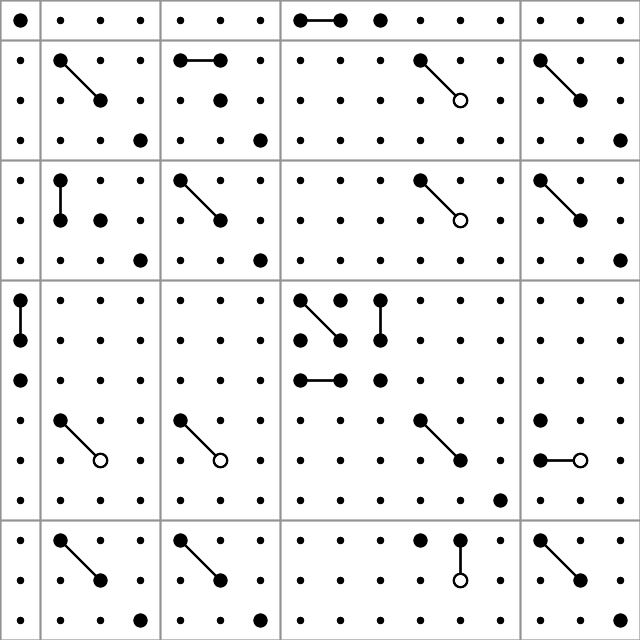So, this is what we’ll be discussing in this post – preparation of such graphs. We’ll be using the PHP module Imagick for ImageMagick functions and objects. On Ubuntu, you can install the package from the command line as:

`sudo apt-get install php5-imagick`

(don’t forget to restart the http server if you’re working via a web interface instead of cli)

Before going further into the graph production, here is a description of producing a random matrix compatible with the given conditions in Octave:

Constructing a sample matrix in Octave

```clear;

# Declare the matrix
T=[];

# == >> === non-zero coefficients ===== 0 =====
# I used the shorcuts and scripting of Vim to easily format the like lines..
non_zero_coeff = [];
non_zero_coeff = [non_zero_coeff; 1 1];
non_zero_coeff = [non_zero_coeff; 1 8];
non_zero_coeff = [non_zero_coeff; 1 9];
non_zero_coeff = [non_zero_coeff; 1 10];
non_zero_coeff = [non_zero_coeff; 2 2];
non_zero_coeff = [non_zero_coeff; 2 5];
non_zero_coeff = [non_zero_coeff; 2 11];
non_zero_coeff = [non_zero_coeff; 2 14];
non_zero_coeff = [non_zero_coeff; 3 3];
non_zero_coeff = [non_zero_coeff; 3 6];
non_zero_coeff = [non_zero_coeff; 3 12];
non_zero_coeff = [non_zero_coeff; 3 15];
non_zero_coeff = [non_zero_coeff; 4 4];
non_zero_coeff = [non_zero_coeff; 4 7];
non_zero_coeff = [non_zero_coeff; 3 12];
non_zero_coeff = [non_zero_coeff; 4 16];
non_zero_coeff = [non_zero_coeff; 5 5];
non_zero_coeff = [non_zero_coeff; 5 11];
non_zero_coeff = [non_zero_coeff; 1 9];
non_zero_coeff = [non_zero_coeff; 5 14];
non_zero_coeff = [non_zero_coeff; 6 6];
non_zero_coeff = [non_zero_coeff; 6 12];
non_zero_coeff = [non_zero_coeff; 1 9];
non_zero_coeff = [non_zero_coeff; 6 15];
non_zero_coeff = [non_zero_coeff; 7 7];
non_zero_coeff = [non_zero_coeff; 7 16];
non_zero_coeff = [non_zero_coeff; 8 8];
non_zero_coeff = [non_zero_coeff; 8 9];
non_zero_coeff = [non_zero_coeff; 8 10];
non_zero_coeff = [non_zero_coeff; 6 15];
non_zero_coeff = [non_zero_coeff; 9 9];
non_zero_coeff = [non_zero_coeff; 9 10];
non_zero_coeff = [non_zero_coeff; 10 10];
non_zero_coeff = [non_zero_coeff; 11 11];
non_zero_coeff = [non_zero_coeff; 11 14];
non_zero_coeff = [non_zero_coeff; 12 12];
non_zero_coeff = [non_zero_coeff; 12 14];
non_zero_coeff = [non_zero_coeff; 13 13];
non_zero_coeff = [non_zero_coeff; 14 14];
non_zero_coeff = [non_zero_coeff; 15 15];
non_zero_coeff = [non_zero_coeff; 16 16];

# Assign random values to the non-zero coefficients:
for i=1:rows(non_zero_coeff)
T(non_zero_coeff(i,1),non_zero_coeff(i,2)) = rand;
endfor
# == << === non-zero coefficients ===== 1 =====

# == >> === Relations ===== 0 =====
T(1,9)=T(1,8);
T(3,3)=T(2,2);
T(2,6)=T(2,5);
T(3,12)=-T(2,11);
T(3,15)=T(2,14);
T(6,6)=T(5,5);
T(6,12)=-T(5,11);
T(6,15)=T(5,14);
T(9,9)=T(8,8);
T(9,10)=T(8,10);
T(12,12)=T(11,11);
T(12,15)=-T(12,14);
T(15,15)=T(14,14);
# == << === Relations ===== 1 =====

# == >> === Symmetry ===== 0 =====
for i=1:rows(T)
for j=i:columns(T)
T(j,i) = T(i,j);
endfor
endfor
# == << === Symmetry ===== 1 =====

# Export the matrix to a file:
save("input.data.txt","T");```

Finding out the relations between the coefficients

Doing a double loop over i=1:rows and j=i:cols, for each of the coefficients in and above the diagonal, taking one as reference (checking if abs(T(i,j))>0 and recording to an array, say “non-zero-coeff-array” if so) and then comparing it with the rest of the elements (that comes after it) with another double loop i2=i:rows and j2=j:cols. If T(i2,j2) is equal to +-T(i,j), we also note this (three values for each i2,j2 equivalency identification: i2,j2 and +/-1) in another array (say “equal-array”).

For example, according to our case the (9,9) element of the non-zero-coeff-array would be TRUE and the same element of the equal-array would be a sub-array holding the values (8,8,1).

Painting on a canvas

Once you have access to the ImageMagick library through Imagick, we can start “painting” a canvas by first defining the canvas (width, height and background color):

``````# Declare the object (/holder)
\$image = new Imagick();

# Define the canvas (size 640x640 pixels with white background)
\$image->newImage( 640, 640, new ImagickPixel( 'white' ) );
``````

Let’s put a circle (filled, black) in the center with a radius of 30 pixels:

``````# Define the circle:
\$circle = new ImagickDraw();
\$circle->setFillColor("#000000");
\$circle->circle(320,320,350,320);

# Add (/register) it to the canvas:
\$image->drawImage(\$circle);

As for the production of the image file, we need to designate it as a PNG image and then export it to a file:
<code># Designate the image type as PNG:
\$image->setImageFormat( "png" );

# Export (/write) it to a file "out.png":
\$fp = fopen("out.png","w");
fwrite(\$fp,\$image);
fclose(\$fp);
``````

This is what you -should- get: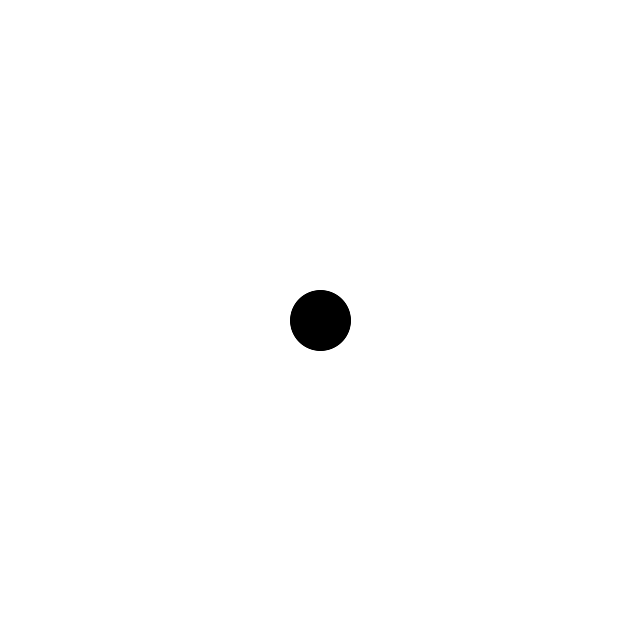not bad for beginning, right? 😉

The circle function has 4 parameters: the (x,y) coordinates of the center and the coordinates of a point that is on the edge of the circle. Let’s draw another circle, this time an unfilled one below this filled one:

``````# Declare the object (/holder)
\$image = new Imagick();

# Define the canvas (size 640x640 pixels with white background)
\$image --->newImage( 640, 640, new ImagickPixel( 'white' ) );

# Define the 1st, filled circle:
\$circle = new ImagickDraw();
\$circle->setFillColor("#000000");
\$circle->circle(320,320,350,320);

# Add (/register) it to the canvas:
\$image->drawImage(\$circle);

# Define the 2nd, unfilled circle:
\$circle = new ImagickDraw();
\$circle->setStrokeColor("#000000");
\$circle->setStrokeWidth(2);
\$circle->setFillColor("none");
\$circle->circle(320,350,350,350);

# Add (/register) it to the canvas:
\$image->drawImage(\$circle);

# Designate the image type as PNG:
\$image->setImageFormat( "png" );

# Export (/write) it to a file "out.png":
\$fp = fopen("out.png","w");
fwrite(\$fp,\$image);
fclose(\$fp);``````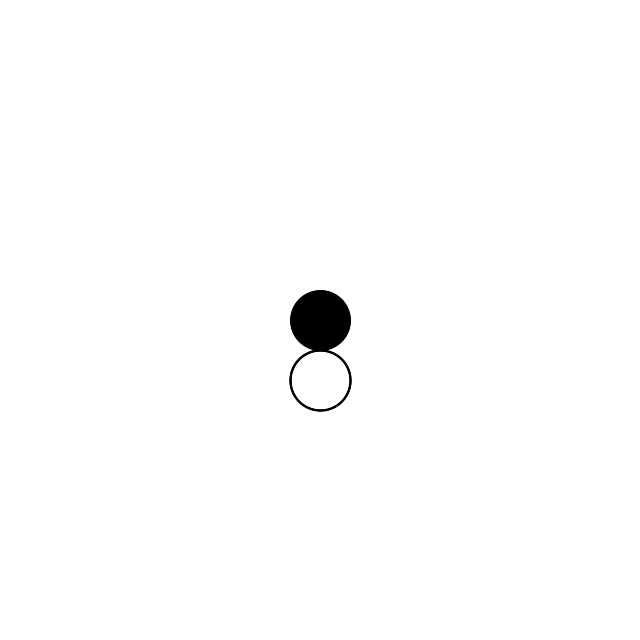The “stroke” methods are responsible for the perimeter and the fillcolor setting to “none” ensures that we have an otherwise invisible circle.

So far, so good.. Let’s add a line that passes between the circles from (120,50) to (520,50):

``````# Define the line:
\$line = new ImagickDraw();
\$line->setStrokeColor("#000000");
\$line->setStrokeWidth(2);
\$line->line(120,350,520,350);
\$image->drawImage(\$line);``````Mapping the rows and cols to x and y

Suppose that we have a 16×16 matrix to be mapped onto a 640×640 canvas. We could map (i=0,j=0) to (x=0,y=0) and (i=16,j=16) to (x=640,y=640) but it wouldn’t be right: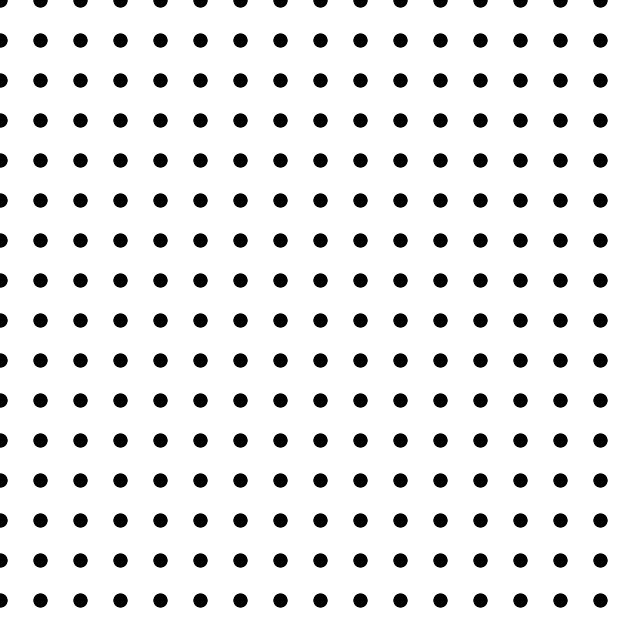doesn’t look right, right? And here’s the code that produced it:

``````# Declare the object (/holder)
\$image = new Imagick();

# Define the canvas (size 640x640 pixels with white background)
\$image--->newImage( 640, 640, new ImagickPixel( 'white' ) );

# Define the 1st, filled circle:
\$dxy = 640 / 16;
for(\$i=1;\$i<=16;\$i++)
{
for(\$j=1;\$j<=16;\$j++)
{
\$circle = new ImagickDraw();
\$circle->setFillColor("#000000");
\$circle->circle(\$dxy*(\$j-1),\$dxy*(\$i-1),\$dxy*(\$j-1)+\$dxy/6,\$dxy*(\$i-1));

# Add (/register) it to the canvas:
\$image->drawImage(\$circle);
}
}

# Designate the image type as PNG:
\$image->setImageFormat( "png" );

# Export (/write) it to a file "out.png":
\$fp = fopen("out.png","w");
fwrite(\$fp,\$image);
fclose(\$fp);
``````

What we need to do is to shift it half of the periodicity in the x & y directions: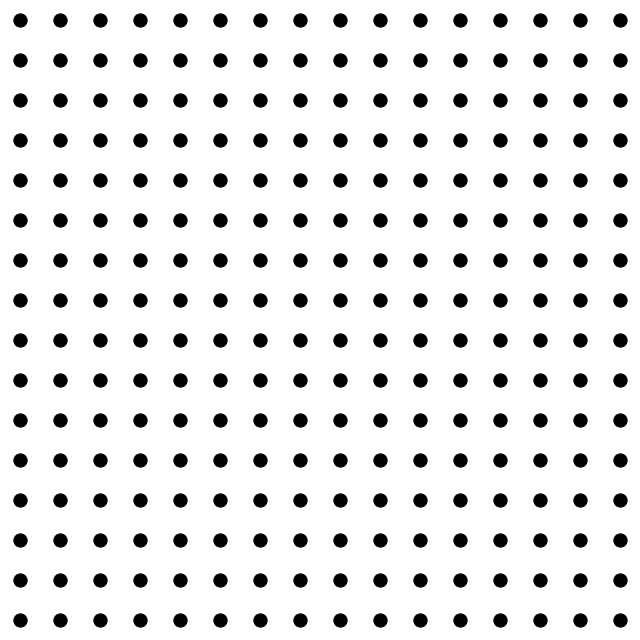That’s more like it — this shift was introduced to the code by changing the coordinate parameters of the circle(s):
`\$circle->circle(\$dxy*(\$j-1/2),\$dxy*(\$i-1/2),\$dxy*(\$j-1/2)+\$dxy/6,\$dxy*(\$i-1/2));`

So, depending on the value (zero/non-zero) of an element of our tensor, we can put it as a big circle (r=\$dxy/6) or a small one (r=\$dxy/12). In addition, if it’s negative, we can have it drawn as an unfilled one (

```\$circle->setStrokeColor("#000000");
\$circle->setStrokeWidth(2);
\$circle->setFillColor("none");```

). We’re almost ready aside from the connecting the related entries!

Connecting the dots

Suppose that we’d like to connect the circles corresponding to the (i1,j1) and the (i2,j2) elements of the tensor. On the canvas, their centers will be given as (\$dxy*(\$j1-1/2),\$dxy*(\$i1-1/2)) and (\$dxy*(\$j2-1/2),\$dxy*(\$i2-1/2)) where \$dxy is the conversion unit of 1 step in the row-col matrix to that of the canvas (for simplicity I assumed a square canvas which is not necessary — then we’d have a \$dx=\$width/\$num_cols and a \$dy=\$height/\$num_rows. Also note that the rows are associated with the height (\$i <-> vertical) and the columns are with the width (\$j <-> horizontal) (that’s why \$j’s come before \$i’s in the center equations).

So, let’s join (11,5) to (9,3): in a 640×640 canvas, (11,5) is centered at ((640/16)*(5-1/2) = 180, (640/16)*(11-1/2) = 420) and similarly (9,3) is centered at (100,340). Let’s put them on the map and connect their centers with a line:

``````
# Declare the object (/holder)
\$image = new Imagick();

# Define the canvas (size 640x640 pixels with white background)
\$image->newImage( 640, 640, new ImagickPixel( 'white' ) );

\$dxy = 640/16; # 40px

# Define the unfilled (11,5) circle:
\$circle = new ImagickDraw();
\$circle->setStrokeColor("#000000");
\$circle->setStrokeWidth(2);
\$circle->setFillColor("none");

# Add (/register) it to the canvas:
\$image->drawImage(\$circle);

# Define the unfilled (9,3) circle:
\$circle = new ImagickDraw();
\$circle->setStrokeColor("#000000");
\$circle->setStrokeWidth(2);
\$circle->setFillColor("none");

# Add (/register) it to the canvas:
\$image->drawImage(\$circle);

# Connect them with a line:
\$line = new ImagickDraw();
\$line->setStrokeColor("#000000");
\$line->setStrokeWidth(2);
\$line->line(180,420,100,340);
\$image->drawImage(\$line);

# Designate the image type as PNG:
\$image->setImageFormat( "png" );

# Export (/write) it to a file "out.png":
\$fp = fopen("out.png","w");
fwrite(\$fp,\$image);
fclose(\$fp);

``````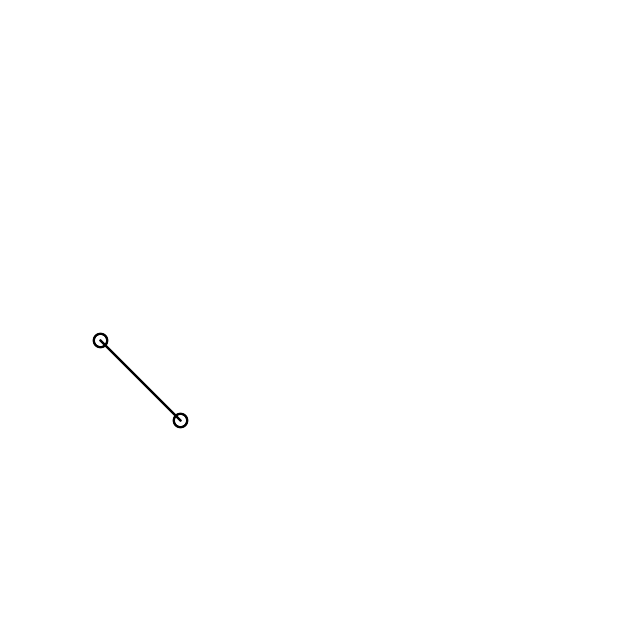Devil is in the details

By now, hopefully you’ve got the idea of how to swing things in your way. In my case, if I spent the 40% of the time doing the things I described, the remaining 60% went in adjusting the line such that it starts on the perimeter of the circle, not from the center of it, i.e.,and this requires the determination of the angle of the line connecting our nodes. The angle of a line passing through two points (x1,y1) & (x2,y2) is given by: arctan[(y2-y1)/(x2-x1)], where the value is nothing but the A of the y=Ax+B line equation, and we need to translate the beginning and the final points of our line in this direction by an amount of a radius:

``````# Find the tangent of the line:
\$tg = (340-420)/(100-180);
\$alpha_deg = \$alpha_radian * 180 / M_PI;

# Connect them with a line:
\$line = new ImagickDraw();
\$line->setStrokeColor("#000000");
\$line->setStrokeWidth(2);
\$image->drawImage(\$line);``````

and that’s more or less all about it!

One picture is worth a thousand words

You can have a sneak peak of the working code via here: http://144.122.31.125/cgi-bin/cryst/programs/tensor_graph/

How to Prepare an Input File for Surface Calculations

Emre S. Tasci

19/07/2013

Abstract

This how-to is intended to guide the reader during the preparation of the input file for an intended surface calculation study. Mainly VESTA software is used to manipulate and transform initial structure file along with some home brewed scripts to do simple operations such as translations.

Description

One of the questions I’m frequently being asked by the students is the preparation of input files for surface calculations (or more accurately, “a practical way” for the purpose). In this text, starting from a structural data file for bulk, the methods to obtain the input file fit for structure calculations will be dealt.

Tools

VESTA [A] is a free 3D visualization program for structural models that also allows editing to some extent. It is available for Windows, MacOS and Linux operating systems.
STRCONVERT [B] is a tool hosted on the Bilbao Crystallographic Server[] (BCS) that offers visualization and also basic editing as well as conversion between various common structural data formats.
TRANSTRU [C] is an another BCS tool that transforms a given structure via the designated transformation matrix.

Procedure

Initial structural data

We start by obtaining the structural data for the unit cell of the material we are interested in. It should contain the unit cell dimensions and the atomic positions. Some common formats in use are: CIF[], VASP[], VESTA[] and BCS formats. Throughout this text, we will be operating on the Fm3mphase of the platinum carbide (PtC), reported by Zaoui & Ferhat[] as reproduced in BCS format in Table 1↓.

225
4.50 4.50 4.50 90. 90. 90.
2
C 1 4a 0 0 0
Pt 1 4b 0.5 0.5 0.5

Table 1 Structural data of Fm3m PtC
These data can be entered directly into VESTA from the form accessed by selecting the “File→New Structure” menu items and then filling the necessary information into the “Unit cell” and “Structure parameters” tabs, as shown in Figures 1↓ and 2↓.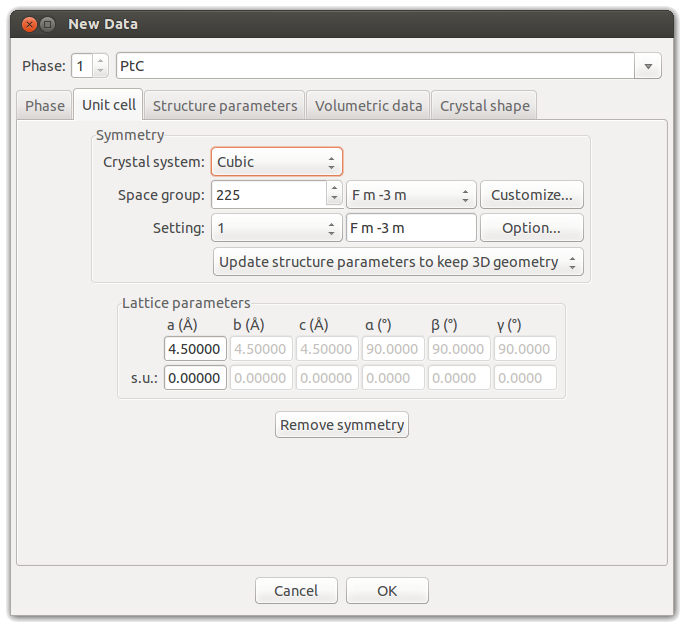Figure 1 VESTA’s “Unit cell” interface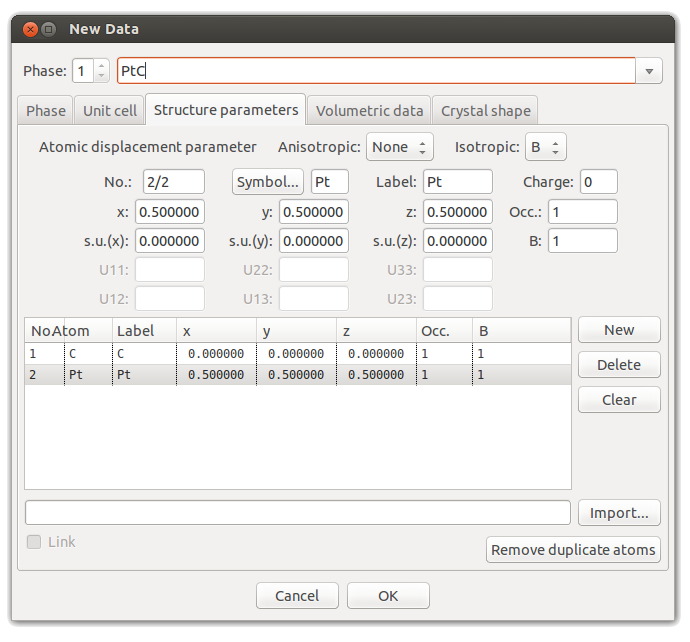Figure 2 VESTA’s “Structure parameters” interface
As a result, we have now defined the Fm3m PtC (Figure 3↓).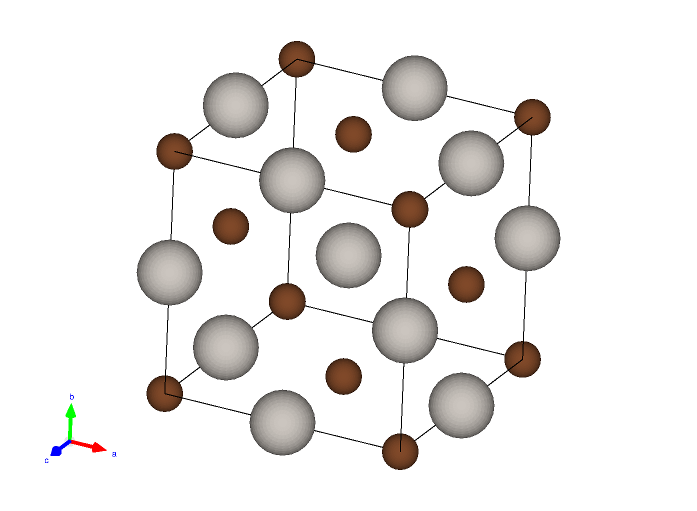Figure 3 Fm3m platinum carbide
An alternative way to introduce the structure would be to directly open its structural data file (obtained from various structure databases such as COD[], ICSD[] or Landolt-Bornstein[] into VESTA.

Constructing the Supercell

A supercell is a collection of unit cells extending in the a,b,c directions. It can easily be constructed using VESTA’s transformation tool. For demonstration purposes, let’s build a 3x3x3 supercell from our cubic PtC cell. Open the “Edit→Edit Data→Unit Cell…” dialog, then click the “Option…” button in the “Setting” row. Then define the transformation matrix as “3a,3b,3c” as shown in Figure 4↓.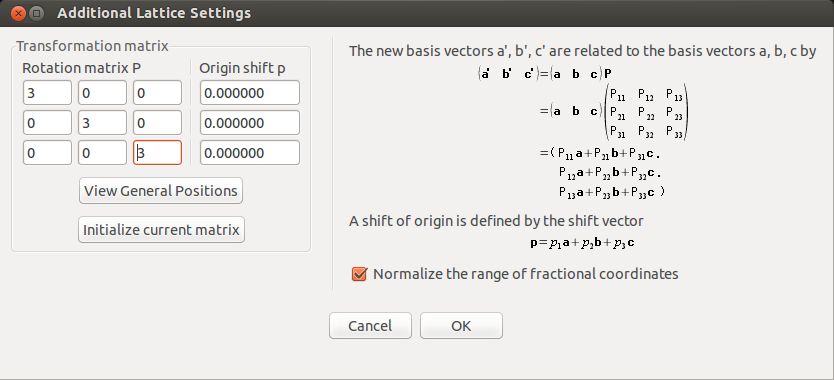Figure 4 Transformation matrix for the supercell
Answer “yes” when the warning for the change of the volume of the unit cell appears, and again click “Yes” to search for additional atoms. After this, click “OK” to exit the dialog. This way we have constructed a supercell of 3x3x3 unit cells as shown in Figure 5↓.Figure 5 A 3x3x3 supercell

,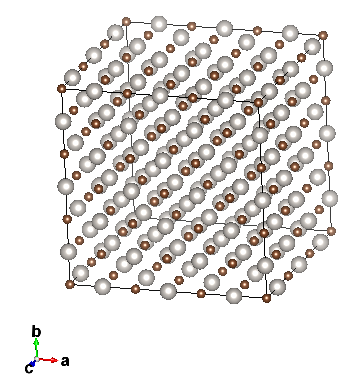Figure 6 Designating the boundaries for the construction of the supercell.
We have built the supercell for a general task but at this stage, it’s not convenient for preparing the surface since we first need to cleave with respect to the necessary lattice plane. So, revert to the conventional unit cell (either by reopening the data file or undoing (CTRL-z) our last action).
Now, populate the space with the conventional cell using the “Boundary” setting under the “Style” tool palette (Figure 6↑). In this case, we are building a 3x3x3 supercell by including the atoms within the -fractional- coordinate range of [0,3] along all the 3 directions (Figure 7↓).Figure 7 (a)Single unit cell (conventional); (b) 3x3x3 supercell
At this stage, the supercell formed is just a mode of display – we haven’t made any solid changes yet. Also, if preferred one can choose how the unit cells will be displayed from the “Properties” setting’s “General” tab.

Lattice Planes

To visualize a given plane with respect to the designated hkl values, open the dialog shown in Figure 8↓ by selecting “Edit→Lattice Planes…” from the menu, then clicking on “New” and entering the intended hkl values, in our example (111) plane.

etasci@metu.edu.tr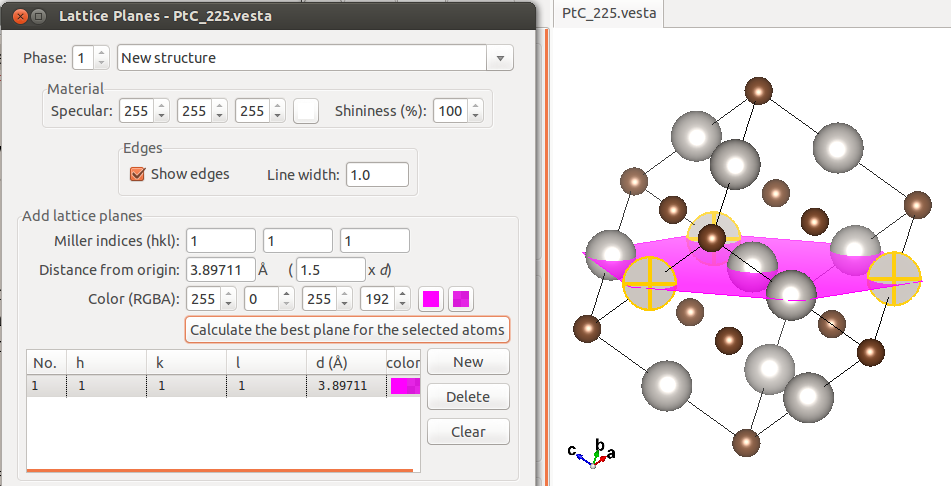Figure 8 Selecting the (111) lattice plane
The plane’s distance to the origin can be specified in terms of Å or interplane distance, as well as selecting 3 or more atoms lying on the plane (multiple selections can be realised by holding down SHIFT while clicking on the atoms) and then clicking on the “Calculate the best plane for the selected atoms” button to have the plane passing from those positions to appear. This is shown in Figure 9↓, done within a 3x3x3 supercell.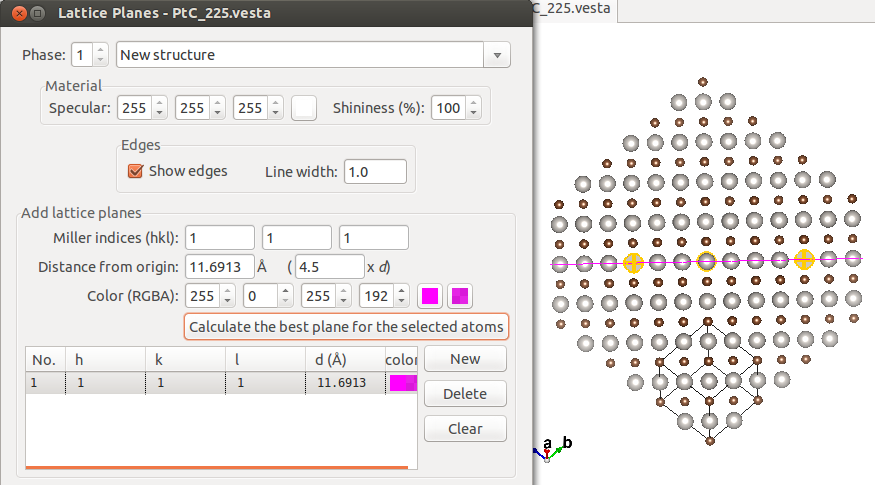Figure 9 Selecting the (111) lattice plane in the 3x3x3 supercell
The reason we have oriented our system so that the normal to the (111) plane is pointing up is to designate this lattice plane as the surface, aligning it with the new c axis for convenience. Therefore we also need to reassign a and b axes, as well. This all comes down to defining a new unit cell. Preserving the periodicities, the new unit cell is traced out by introducing additional lattice planes. These new unit cells are not unique, as long as one preserves the periodicities in the 3 axial directions, they can be taken as large and in any convenient shape as allowed. A rectangular shaped such a unit cell, along with the outlying lattice plane parameters is presented in Figure 10↓ (the boundaries have been increased to [-2,5] in each direction for practicality).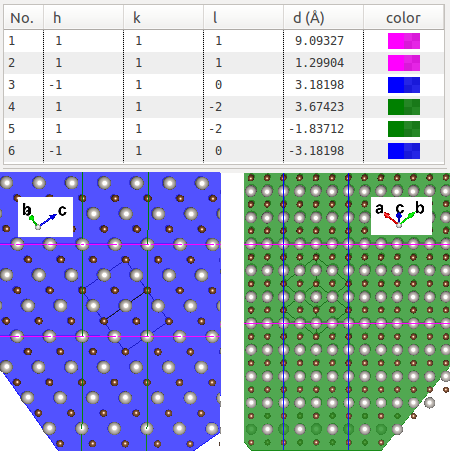Figure 10 The new unit cell marked out by the lattice plane “boundaries”

Trimming the new unit cell

The next procedure is simple and direct: remove all the atoms outside the new unit cell. You can use the “Select” tool from the icons on the left (the 2nd from top) or the shortcut key “s”. The “trimmed” new unit cell is shown in Figure 11↓, take note of the periodicity in each section. It should be noted that, this procedure is only for deducing the transformation matrix, which we’ll be deriving in the next subsection: other than that, since it’s still defined by the “old” lattice vectors, it’s not stackable to yield the correct periodicity so we’ll be fixing that.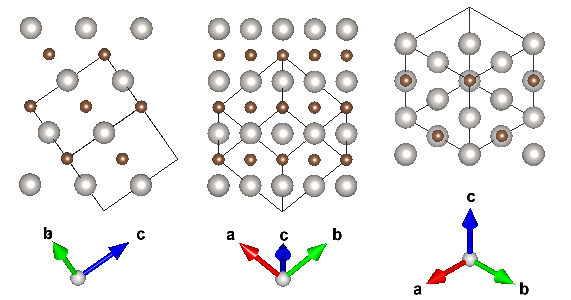Figure 11 Filtered new unit cell with the original cell’s lattice vectors

Transforming into the new unit cell

Now that we have the new unit cell, it is time to transform the unit cell vectors to comply with the new unit cell. For the purpose of obtaining the transformation matrix (which actually is the matrix that relates the new ones with the old ones), we will need the coordinates of the 4 atoms on the edges. Such a set of 4 selected atoms are shown in Figure 12↓. These atoms’ fractional coordinates are as listed in Table 2↓ (upon selecting an atom, VESTA displays its coordinates in the information panel below the visualization).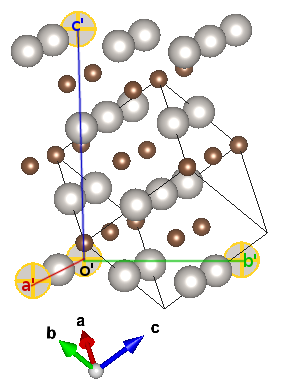Figure 12 The reference points for the new axe1s

 Label a’ b’ c’ o’ a 0 1/2 2 1 b 1 -1/2 1 0 c -1/2 1/2 1/2 -1/2
Table 2 Reference atoms’ fractional coordinates
Taking o’ as our new origin, the new lattice vectors in terms of the previous ones become (as we subtract the “origin’s” coordinates):
a’ = -a+b
b’ = -1/2a-1/2b+c
c’ = a+b+c
Therefore our transformation matrix is:
− 1 − 121 1 − 121 011
(i.e., “-a+b,-1/2a-1/2b+c,a+b+c” — the coefficients are read in columns)
Transforming the initial cell with respect to this matrix is pretty straight forward, in the same manner we exercised in subsection 3.2↑, “Constructing the supercell”, i.e., “Edit→Edit Data→Unit Cell..”, then “Remove symmetry” and “Option…” and introducing the transformation matrix. A further translation (origin shift) of (0,0,1/2) is necessary (the transformation matrix being “-a+b,-1/2a-1/2b+c,a+b+c+1/2”) if you want the transformed structure look exactly like the one shown in Figure 11↑.

A Word of caution

Due to a bug, present in VESTA, you might end with a transformed matrix with missing atoms (as shown in Figure – compare it to the middle and rightmost segments of Figure 11↑). For this reason, always make sure you check your resulting unit cell thoroughly! (This bug has recently been fixed and will be patched in the next version (3.1.7)-personal communication with K.Momma)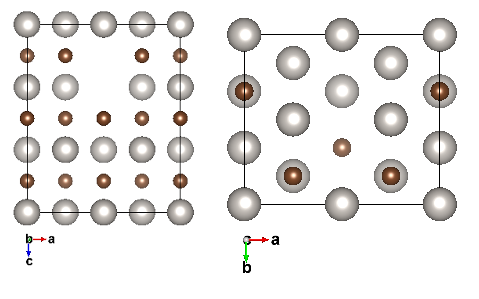Figure 13 The transformed structure with missing atoms
To overcome this problem, one can conduct the transformation via the TRANSTRU [E] tool of the Bilbao Crystallographic Server. Enter your structural data (either as a CIF or by definition into the text box), select “Transform structure to a subgroup basis”, in the next window enter “1” as the “Low symmetry Space Group ITA number” (1 corresponding to the P1 symmetry group) and the transformation matrix either in abc notation (“-a+b,-1/2a-1/2b+c,a+b+c+1/2”) or by directly entering the matrix form (both are filled in the screenshot taken in Figure 14↓).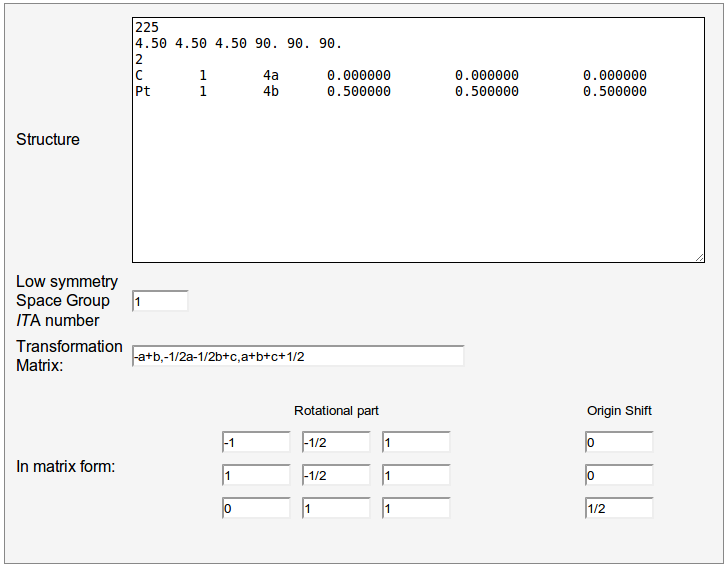Figure 14 TRANSTRU input form
In the result page export the transformed structure data (“Low symmetry structure data”) to CIF format by clicking on the corresponding button and open it in VESTA. This way all the atoms in the new unit cell will have been included.

Final Touch

The last thing remains is introducing a vacuum area above the surface. For this purpose we switch from fractional coordinates to Cartesian coordinates, so when the cell boundaries are altered, the atomic positions will not change as a side result. VASP format supports both notations so we export our structural data into VASP format (“File→Export Data…”, select “VASP” as the file format, then opt for “Write atomic coordinates as: Cartesian coordinates”).
After the VASP file with the atomic coordinates written in Cartesian format is formed, open it with an editor (the contents are displayed in Table3↓).

generated_by_bilbao_crystallographic_server

1.0

6.3639612198 0.0000000000 0.0000000000

0.0000000000 5.5113520622 0.0000000000

0.0000000000 0.0000000000 7.7942290306

C Pt

12 12

Cartesian

0.000000000 3.674253214 6.495164688

0.000000000 1.837099013 1.299064110

3.181980610 1.837099013 1.299064110

1.590990305 0.918577018 6.495164688

4.772970915 4.592774880 1.299064110

1.590990305 4.592774880 1.299064110

4.772970915 0.918577018 6.495164688

0.000000000 0.000000000 3.897114515

3.181980610 0.000000000 3.897114515

4.772970915 2.755676031 3.897114515

1.590990305 2.755676031 3.897114515

3.181980610 3.674253214 6.495164688

0.000000000 3.674253214 2.598050405

1.590990305 0.918577018 2.598050405

4.772970915 0.918577018 2.598050405

1.590990305 4.592774880 5.196178858

3.181980610 1.837099013 5.196178858

0.000000000 1.837099013 5.196178858

4.772970915 4.592774880 5.196178858

0.000000000 0.000000000 0.000000000

3.181980610 3.674253214 2.598050405

3.181980610 0.000000000 0.000000000

4.772970915 2.755676031 0.000000000

1.590990305 2.755676031 0.000000000
Table 3 The constructed (111) oriented VASP file’s contents
Directly edit and increase the c-lattice length from the given value (7.7942 Å in our case) to a sufficiently high value (e.g., 25.0000 Å) and save it. Now when you open it back in VESTA, the surface with its vacuum should be there as in Figure 15↓.Figure 15 Prepared surface with vacuum
At this point, there might be a couple of questions that come to mind: What are those Pt atoms doing at the top of the unit cell? Why are the C atoms positioned above the batch instead of the Pt atoms?
The answer to these kind of questions is simple: Periodicity. By tiling the unit cell in the c-direction using the “Boundary…” option and designating our range of coordinates for the {x,y,z}-directions from 0 to 3, we obtain the system shown in Figure 16↓.Figure 16 New unit cell with periodicity applied
From the periodicity, in fractional coordinates, f|z = 0 = f|z = 1, meaning the atoms at the base and at the very top of the unit cell are the same (or “equivalent” from the interpretational side). If we had wanted the Pt atoms to be the ones forming the surface, then we would have made the transformation with the “-a+b,-1/2a-1/2b+c,a+b+c” matrix instead of “-a+b,-1/2a-1/2b+c,a+b+c+1/2”, so the half shift would amount the change in the order, and then increase the c-lattice parameter size in the Cartesian coordinates notation (i.e., in the VASP file). The result of such a transformation is given in Figure 17↓.Figure 17 Alternative surface with the Pt atoms on the top (Left: prior to vacuum introduction; Center: with vacuum; Right: tiled in periodicity)

Alternative Ways

In this work, a complete treatment of preparing a surface in silico is proposed. There are surely more direct and automated tools that achieve the same task. It has been brought to our attention that the commercial software package Accelrys Materials Studio [F] has an integrated tool called “Cleave Surface” which exactly serves for the same purpose discussed in this work. Since it is propriety software, it will not be further described here and we encourage the user to benefit from freely accessible software whenever possible.
Also, it is always to compare your resulting surface with that of an alternate one obtained using another tool. In this sense, Surface Explorer [G] is very useful in visualizing how the surface will look, eventhough its export capabilities are limited.
Chapter 4 (“DFT Calculations for Solid Surfaces”) of Zhang’s Ph.D. thesis[] contains very fruitful discussions on possible ways to incorporate the surfaces in computations.
It is the author’s intention to soon implement such an automated tool for constructing surfaces from bulk data, integrated within the Bilbao Crystallographic Server’s framework of tools.

Conclusion

Preparing a surface fit for atomic & molecular calculations can be tedious and tiresome. In this work, we have tried to guide the reader in a step-by-step procedure, sometimes wandering off from the direct path to show the mechanisms and reasons behind the usually taken on an “as-it-is” basis, without being pondered upon. This way, we hope that the techniques acquired throughout the text will be used in conjunction with other related problems in the future. A “walkthru” for the case studied ((111) PtC surface preparation) is included as Appendix to provide a quick consultation in the future.

Acknowledgements

This work is the result of the inquiries by the Ph.D. students M. Gökhan Şensoy and O. Karaca Orhan. If they hadn’t asked a “practical way” to construct surfaces, I wouldn’t have sought a way. Since I don’t have much experience with surfaces, I turned to my dear friends Rengin Peköz and O. Barış Malcıoğlu for help, whom enlightened me with their experiences on the issue.

Appendix: Walkthru for PtC (111) Surface

1. Open VESTA, (“File→New Structure”)
1. “Unit Cell”:
1. Space Group: 225 (F m -3 m)
2. Lattice parameter a: 4.5 Å
2. “Structure Parameters”:
1. New→Symbol:C; Label:C; x,y,z=0.0
2. New→Symbol:Pt; Label:Pt; x,y,z=0.5
3. “Boundary..”
1. x(min)=y(min)=z(min)=-2
2. x(max)=y(max)=z(max)=5
4. “Edit→Lattice Planes…”
1. (hkl)=(111); d(Å)=9.09327
2. (hkl)=(111); d(Å)=1.29904
3. (hkl)=(-110); d(Å)=3.18198
4. (hkl)=(-110); d(Å)=-3.18198
5. (hkl)=(11-2); d(Å)=3.67423
6. (hkl)=(11-2); d(Å)=-4.59279
(compare with Figure 10↑)
5. Select (“Select tool” – shortcut “s” key) and delete (shortcut “Del” key) all the atoms lying out of the area designated by the lattice planes.
6. Select an “origin-atom” of a corner and the 3 “axe-atoms” in the edge of each direction of the unit cell, write down their fractional coordinates
1. o(1,0,-1/2)
2. a(0,1,-1/2)
3. b(1/2,-1/2,1/2)
4. c(2,1,1/2)
7. Subtract the origin-atom coordinates from the rest and construct the transformation matrix accordingly
P =  − 1 − 121 1 − 121 011
8. Transform the initial unit cell with this matrix via TRANSTRU (http://www.cryst.ehu.es/cryst/transtru.html) (Figure 14↑)
9. Save the resulting (low symmetry) structure as CIF, open it in VESTA, export it to VASP, select “Cartesian coordinates”
10. Open the VASP file in an editor, increase the c-lattice parameter to a big value (e.g. 25.000) to introduce vacuum. Save it and open it in VESTA.

References

 Mois Ilia Aroyo, Juan Manuel Perez-Mato, Cesar Capillas, Eli Kroumova, Svetoslav Ivantchev, Gotzon Madariaga, Asen Kirov, and Hans Wondratschek. Bilbao crystallographic server: I. databases and crystallographic computing programs. Zeitschrift für Kristallographie, 221(1):1527, 01 2006. doi: 10.1524/zkri.2006.221.1.15.

 Mois I. Aroyo, Asen Kirov, Cesar Capillas, J. M. Perez-Mato, and Hans Wondratschek. Bilbao crystallographic server. ii. representations of crystallographic point groups and space groups. Acta Crystallographica Section A, 62(2):115128, Mar 2006. http://dx.doi.org/10.1107/S0108767305040286

 M I Aroyo, J M Perez-Mato, D Orobengoa, E Tasci, G De La Flor, and A Kirov. Crystallography online: Bilbao crystallographic server. Bulg Chem Commun, 43(2):183197, 2011.

 S. R. Hall, F. H. Allen, and I. D. Brown. The crystallographic information le (cif): a new standard archive file for crystallography. Acta Crystallographica Section A, 47(6):655685, Nov 1991.

 G. Kresse and J. Furthmüller. Ecient iterative schemes for ab initio total-energy calculations using a plane-wave basis set. Phys. Rev. B, 54:1116911186, Oct 1996.

 Koichi Momma and Fujio Izumi. VESTA3 for three-dimensional visualization of crystal, volumetric and morphol

 A Zaoui and M Ferhat. Dynamical stability and high pressure phases of platinum carbide. Solid State Communi

 Saulius Grazulis, Adriana Daskevic, Andrius Merkys, Daniel Chateigner, Luca Lutterotti, Miguel Quirós, Nadezhda R. Serebryanaya, Peter Moeck, Robert T. Downs, and Armel Le Bail. Crystallography open database (cod): an open-access collection of crystal structures and platform for world-wide collaboration. Nucleic

 Alec Belsky, Mariette Hellenbrandt, Vicky Lynn Karen, and Peter Luksch. New developments in the inorganic crystal structure database (icsd): accessibility in support of materials research and design. Acta Crystallographica

 Springer, editor. The Landolt-Boernstein Database (http://www.springermaterials.com/navigation/). Springer Materials.

 Yongsheng Zhang. First-principles statistical mechanics approach to step decoration at solid surfaces . PhD thesis,

❬✶❪ ▼♦✐s ■❧✐❛ ❆r♦2♦✱ ❏✉❛♥ ▼❛♥✉❡❧ P❡r❡3✲▼❛t♦✱ ❈❡s❛r ❈❛♣✐❧❧❛s✱ ❊❧✐ ❑r♦✉♠♦✈❛✱ ❙✈❡t♦s❧❛✈ ■✈❛♥t❝❤❡✈✱ ●♦t3♦♥ ▼❛❞❛r✐❛❣❛✱
❆s❡♥ ❑✐r♦✈✱ ❛♥❞ ❍❛♥s ❲♦♥❞r❛ts❝❤❡❦✳ ❇✐❧❜❛♦ ❝r2st❛❧❧♦❣r❛♣❤✐❝ s❡r✈❡r✿ ■✳ ❞❛t❛❜❛s❡s ❛♥❞ ❝r2st❛❧❧♦❣r❛♣❤✐❝ ❝♦♠♣✉t✐♥❣
♣r♦❣r❛♠s✳ ❩❡✐ts❝❤r✐❢t ❢ür ❑r✐st❛❧❧♦❣r❛♣❤✐❡✱ ✷✷✶✭✶✮✿✶✺✕✷✼✱ ✵✶ ✷✵✵✻✳ ❞♦✐✿ ✶✵✳✶✺✷✹✴3❦r✐✳✷✵✵✻✳✷✷✶✳✶✳✶✺✳
❬✷❪ ▼♦✐s ■✳ ❆r♦2♦✱ ❆s❡♥ ❑✐r♦✈✱ ❈❡s❛r ❈❛♣✐❧❧❛s✱ ❏✳ ▼✳ P❡r❡3✲▼❛t♦✱ ❛♥❞ ❍❛♥s ❲♦♥❞r❛ts❝❤❡❦✳ ❇✐❧❜❛♦ ❝r2st❛❧❧♦❣r❛♣❤✐❝
s❡r✈❡r✳ ✐✐✳ r❡♣r❡s❡♥t❛t✐♦♥s ♦❢ ❝r2st❛❧❧♦❣r❛♣❤✐❝ ♣♦✐♥t ❣r♦✉♣s ❛♥❞ s♣❛❝❡ ❣r♦✉♣s✳ ❆❝t❛ ❈r2st❛❧❧♦❣r❛♣❤✐❝❛ ❙❡❝t✐♦♥ ❆✱
✻✷✭✷✮✿✶✶✺✕✶✷✽✱ ▼❛r ✷✵✵✻✳
❬✸❪ ▼ ■ ❆r♦2♦✱ ❏ ▼ P❡r❡3✲▼❛t♦✱ ❉ ❖r♦❜❡♥❣♦❛✱ ❊ ❚❛s❝✐✱ ● ❉❡ ▲❛ ❋❧♦r✱ ❛♥❞ ❆ ❑✐r♦✈✳ ❈r2st❛❧❧♦❣r❛♣❤2 ♦♥❧✐♥❡✿ ❇✐❧❜❛♦
❝r2st❛❧❧♦❣r❛♣❤✐❝ s❡r✈❡r✳ ❇✉❧❣ ❈❤❡♠ ❈♦♠♠✉♥✱ ✹✸✭✷✮✿✶✽✸✕✶✾✼✱ ✷✵✶✶✳
❬✹❪ ❙✳ ❘✳ ❍❛❧❧✱ ❋✳ ❍✳ ❆❧❧❡♥✱ ❛♥❞ ■✳ ❉✳ ❇r♦✇♥✳ ❚❤❡ ❝r2st❛❧❧♦❣r❛♣❤✐❝ ✐♥❢♦r♠❛t✐♦♥ ✜❧❡ ✭❝✐❢✮✿ ❛ ♥❡✇ st❛♥❞❛r❞ ❛r❝❤✐✈❡ ✜❧❡
❢♦r ❝r2st❛❧❧♦❣r❛♣❤2✳ ❆❝t❛ ❈r2st❛❧❧♦❣r❛♣❤✐❝❛ ❙❡❝t✐♦♥ ❆✱ ✹✼✭✻✮✿✻✺✺✕✻✽✺✱ ◆♦✈ ✶✾✾✶✳❍❖❲ ❚❖ P❘❊P❆❘❊ ❆◆ ■◆P❯❚ ❋■▲❊ ❋❖❘ ❙❯❘❋❆❈❊ ❈❆▲❈❯▲❆❚■❖◆❙
✶✻
❬✺❪ ●✳ ❑r❡ss❡ ❛♥❞ ❏✳ ❋✉rt❤♠ü❧❧❡r✳ ❊✣❝✐❡♥t ✐t❡r❛t✐✈❡ s❝❤❡♠❡s ❢♦r ❛❜ ✐♥✐t✐♦ t♦t❛❧✲❡♥❡r❣2 ❝❛❧❝✉❧❛t✐♦♥s ✉s✐♥❣ ❛ ♣❧❛♥❡✲✇❛✈❡
❜❛s✐s s❡t✳ P❤2s✳ ❘❡✈✳ ❇✱ ✺✹✿✶✶✶✻✾✕✶✶✶✽✻✱ ❖❝t ✶✾✾✻✳
❬✻❪ ❑♦✐❝❤✐ ▼♦♠♠❛ ❛♥❞ ❋✉❥✐♦ ■3✉♠✐✳ ❱❊❙❚❆✸ ❢♦r t❤r❡❡✲❞✐♠❡♥s✐♦♥❛❧ ✈✐s✉❛❧✐3❛t✐♦♥ ♦❢ ❝r2st❛❧✱ ✈♦❧✉♠❡tr✐❝ ❛♥❞ ♠♦r♣❤♦❧✲
♦❣2 ❞❛t❛✳ ❏♦✉r♥❛❧ ♦❢ ❆♣♣❧✐❡❞ ❈r2st❛❧❧♦❣r❛♣❤2✱ ✹✹✭✻✮✿✶✷✼✷✕✶✷✼✻✱ ❉❡❝ ✷✵✶✶✳
❬✼❪ ❆ ❩❛♦✉✐ ❛♥❞ ▼ ❋❡r❤❛t✳ ❉2♥❛♠✐❝❛❧ st❛❜✐❧✐t2 ❛♥❞ ❤✐❣❤ ♣r❡ss✉r❡ ♣❤❛s❡s ♦❢ ♣❧❛t✐♥✉♠ ❝❛r❜✐❞❡✳ ❙♦❧✐❞ ❙t❛t❡ ❈♦♠♠✉♥✐✲
❝❛t✐♦♥s✱ ✶✺✶✭✶✷✮✿✽✻✼✕✽✻✾✱ ✻ ✷✵✶✶✳
❬✽❪ ❙❛✉❧✐✉s ●r❛➸✉❧✐s✱ ❆❞r✐❛♥❛ ❉❛➨❦❡✈✐↔✱ ❆♥❞r✐✉s ▼❡r❦2s✱ ❉❛♥✐❡❧ ❈❤❛t❡✐❣♥❡r✱ ▲✉❝❛ ▲✉tt❡r♦tt✐✱ ▼✐❣✉❡❧ ◗✉✐rós✱
◆❛❞❡3❤❞❛ ❘✳ ❙❡r❡❜r2❛♥❛2❛✱ P❡t❡r ▼♦❡❝❦✱ ❘♦❜❡rt ❚✳ ❉♦✇♥s✱ ❛♥❞ ❆r♠❡❧ ▲❡ ❇❛✐❧✳ ❈r2st❛❧❧♦❣r❛♣❤2 ♦♣❡♥ ❞❛t❛✲
❜❛s❡ ✭❝♦❞✮✿ ❛♥ ♦♣❡♥✲❛❝❝❡ss ❝♦❧❧❡❝t✐♦♥ ♦❢ ❝r2st❛❧ str✉❝t✉r❡s ❛♥❞ ♣❧❛t❢♦r♠ ❢♦r ✇♦r❧❞✲✇✐❞❡ ❝♦❧❧❛❜♦r❛t✐♦♥✳ ◆✉❝❧❡✐❝
❆❝✐❞s ❘❡s❡❛r❝❤✱ ✹✵✭❉✶✮✿❉✹✷✵✕❉✹✷✼✱ ✷✵✶✷✳
❬✾❪ ❆❧❡❝ ❇❡❧s❦2✱ ▼❛r✐❡tt❡ ❍❡❧❧❡♥❜r❛♥❞t✱ ❱✐❝❦2 ▲2♥♥ ❑❛r❡♥✱ ❛♥❞ P❡t❡r ▲✉❦s❝❤✳ ◆❡✇ ❞❡✈❡❧♦♣♠❡♥ts ✐♥ t❤❡ ✐♥♦r❣❛♥✐❝
❝r2st❛❧ str✉❝t✉r❡ ❞❛t❛❜❛s❡ ✭✐❝s❞✮✿ ❛❝❝❡ss✐❜✐❧✐t2 ✐♥ s✉♣♣♦rt ♦❢ ♠❛t❡r✐❛❧s r❡s❡❛r❝❤ ❛♥❞ ❞❡s✐❣♥✳ ❆❝t❛ ❈r2st❛❧❧♦❣r❛♣❤✐❝❛
❙❡❝t✐♦♥ ❇✱ ✺✽✭✸ P❛rt ✶✮✿✸✻✹✕✸✻✾✱ ❏✉♥ ✷✵✵✷✳
❬✶✵❪ ❙♣r✐♥❣❡r✱ ❡❞✐t♦r✳ ❚❤❡ ▲❛♥❞♦❧t✲❇♦❡r♥st❡✐♥ ❉❛t❛❜❛s❡ ✭❤tt♣✿✴✴✇✇✇✳s♣r✐♥❣❡r♠❛t❡r✐❛❧s✳❝♦♠✴♥❛✈✐❣❛t✐♦♥✴✮✳ ❙♣r✐♥❣❡r
▼❛t❡r✐❛❧s✳
❬✶✶❪ ❨♦♥❣s❤❡♥❣ ❩❤❛♥❣✳ ❋✐rst✲♣r✐♥❝✐♣❧❡s st❛t✐st✐❝❛❧ ♠❡❝❤❛♥✐❝s ❛♣♣r♦❛❝❤ t♦ st❡♣ ❞❡❝♦r❛t✐♦♥ ❛t s♦❧✐❞ s✉r❢❛❝❡s✳ P❤❉ t❤❡s✐s✱
❋r❡✐ ❯♥✐✈❡rs✐t❛t ❇❡r❧✐♥✱ ✷✵✵✽✳

Document generated by eLyXer 1.2.3 (2011-08-31) on 2013-09-11T11:44:36.446114

Meanwhile…

August 7, 2012 Posted by Emre S. Tasci

… the Imperial Tie-Fighters kept on coming… (aka Structure Data Converter & Editor + Visualizer with full support for magnetic space groups)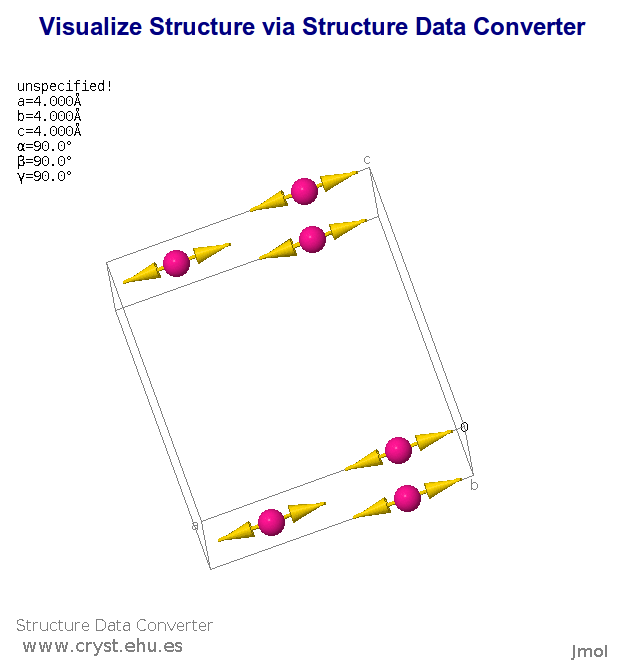Quantum Espresso meets bash : Automated running for various values

March 13, 2012 Posted by Emre S. Tasci

Now that we can include comments in our input files and submit our jobs in a tidied up way, it’s time to automatize the “convergence search” process. For this, we’ll be using the same diamond example of the previous entry.

Suppose that we want to calculate the energies corresponding to various values of the lattice parameter a, ranging from 3.2 to 4, incrementing by 2: 3.2, 3.4, … , 4.0

Incrementation:
In bash, you can define a for loop to handle this:

```for (( i = 1; i &lt;= 9; i++ ))
do
echo \$i;
done

1
2
3
4
5
6
7
8
9```

But to work with rational numbers, things get a little bit complex:
```for (( i = 1; i &lt;= 5; i++ ))
do
val=\$(awk "BEGIN {print 3+\$i/5 }")
echo \$val;
done

3.2
3.4
3.6
3.8
4```

Alas, it is still not very practical to everytime calculate the necessary values to produce our intended range. This is where the mighty Octave once again comes to the rescue: [A:I:B] in Octave defines a range that starts from A, and continues by incrementing by I until B is reached (or exceeded). So, here is our example in Octave:
```octave:1&gt; [3.2:0.2:4]
ans =

3.2000   3.4000   3.6000   3.8000   4.0000```

You can generate temporary input scripts for Octave but better yet, you can also feed simple scripts directly to Octave:
```sururi@husniya:~/shared/qe/diamond_tutorial\$ echo "[3.2:0.2:4]"|octave -q
ans =

3.2000   3.4000   3.6000   3.8000   4.0000```

The “-q” parameter causes Octave to run in “quiet” mode, so we just feed the range via pipe to Octave and will harvest the output. In general, we’d like to keep the “ans =” and the blank lines out, and then, when there are more than 1 line’s worth of output, Octave puts the “Column” information as in:
```octave:4&gt; [3:0.1:4]
ans =

Columns 1 through 9:

3.0000   3.1000   3.2000   3.3000   3.4000   3.5000   3.6000   3.7000   3.8000

Columns 10 and 11:

3.9000   4.0000```

all these nuisances can go away via a simple “grep -v” (with the ‘v’ parameter inverting the filter criteria):
```sururi@vala:/tmp\$ echo "[3.2:0.1:4]"|octave -q|sed -n "2,1000p"| grep -v "Column"

3.2000   3.3000   3.4000   3.5000   3.6000   3.7000   3.8000   3.9000

4.0000

sururi@vala:/tmp\$ echo "[3.2:0.1:4]"|octave -q|sed -n "2,1000p"| grep -v "Column"|grep -v -e "^\$"
3.2000   3.3000   3.4000   3.5000   3.6000   3.7000   3.8000   3.9000
4.0000```

Replacement:
Now that we have the variable’s changing values, how are we gonna implement it to the input script? By search and replace. Suppose, we have a template in which the variable’s value is specified by “#REPLACE#”, all we need to do is to “sed” the file:
`cat diamond.scf.in | sed "s:#REPLACE#:\$a:g"&gt;tmp_\$a.in`

where “\$a” is the current value in the iteration.

Parsing the Energy:
We submit this job via mpirun (in our case via mpi-pw.x of the previous post) and parse the output for the final energy:

`grep -e ! tmp_\$a.out|tail -n1|awk '{print \$(NF-1)}'`

For each iteration we thus obtain the energy for our \$a value, so we put them together in two columns into a data file I’d like to call “E_vs_\$a.txt”.

The Script:
Putting all these together, here is the script in its full glory:

```#!/bin/bash

# Takes in the template Quantum Espresso input file, runs it iteratively
# changing the #REPLACE# parameters iteratively with the range elements,
# grepping the energy and putting it along with the current value of the
# #REPLACE# parameter.
#
# Emre S. Tasci, 03/2012

# Example: Suppose that the file "diamond.scf.in" contains the following line:
#   A = #REPLACE#
# Calling "qe-opti.sh diamond.scf.in a 3.2 0.2 4.2" would cause that line to be modified as
#   A = 3.2
# and submitted, followed by
#   A = 3.4, 3.6, .., 4.2 in the sequential runs.
#
# at the end, having constructed the E_vs_a.txt file.

if [ \$# -ne 5 ]
then
echo "Usage: qe-opti.sh infile_template.in variable_name initial_value increment     final_value"
echo "Example: qe-opti.sh diamond.scf.in a 3.2 0.2 4.2"
echo -e "\nNeeds octave to be accessible via \"octave\" command for the range to     work"
echo -e "\nEmre S. Tasci, 03/2012"
exit
fi

logext=".txt"
logfilename=E_vs_\$2\$logext
rm -f \$logfilename

range=\$(echo "[\$3:\$4:\$5]"|octave -q|sed -n "2,1000p"|grep -vr "^\$"|grep -v "Column")
for a in \$range
do
# echo \$a
cat \$1|sed "s:#REPLACE#:\$a:g"&gt;tmp_\$a.in
/home/sururi/bin/mpi-pw.x 4 tmp_\$a.in
energ=\$(grep -e ! tmp_\$a.out|tail -n1|awk '{print \$(NF-1)}')
# echo \$energ
echo -e \$a "    " \$energ &gt;&gt; \$logfilename
echo -e \$a "    " \$energ
done

echo "Results stored in: "\$logfilename```

Action:
Modify the sample input from the previous post so that it’s lattice parameter A is designated as something like “A = #REPLACE#”, as in:
```sururi@bebop:~/shared/qe/diamond_tutorial\$ grep "REPLACE" -B2 -A2 diamond.scf.in
# a,b,c in Angstrom =======
#A = 3.56712
A = #REPLACE#
B = 2.49
C = 2.49```

Then call the qe-opti.sh script with the related parameters:
```sururi@bebop:~/shared/qe/diamond_tutorial\$ qe-opti.sh diamond.scf.in a 3.2 0.2 4.2
Running: mpirun -n 4 /usr/share/espresso-4.3.2/bin/pw.x &lt; tmp_3.2000.in &gt; tmp_3.2000.out

Start : Tue Mar 13 23:21:07 CET 2012
Finish: Tue Mar 13 23:21:08 CET 2012
Elapsed time:0:00:01

3.2000      -22.55859979
Running: mpirun -n 4 /usr/share/espresso-4.3.2/bin/pw.x &lt; tmp_3.4000.in &gt; tmp_3.4000.out

Start : Tue Mar 13 23:21:08 CET 2012
Finish: Tue Mar 13 23:21:09 CET 2012
Elapsed time:0:00:01

3.4000      -22.67717405
Running: mpirun -n 4 /usr/share/espresso-4.3.2/bin/pw.x &lt; tmp_3.6000.in &gt; tmp_3.6000.out

Start : Tue Mar 13 23:21:09 CET 2012
Finish: Tue Mar 13 23:21:10 CET 2012
Elapsed time:0:00:01

3.6000      -22.69824702
Running: mpirun -n 4 /usr/share/espresso-4.3.2/bin/pw.x &lt; tmp_3.8000.in &gt; tmp_3.8000.out

Start : Tue Mar 13 23:21:10 CET 2012
Finish: Tue Mar 13 23:21:11 CET 2012
Elapsed time:0:00:01

3.8000      -22.65805473
Running: mpirun -n 4 /usr/share/espresso-4.3.2/bin/pw.x &lt; tmp_4.0000.in &gt; tmp_4.0000.out

Start : Tue Mar 13 23:21:11 CET 2012
Finish: Tue Mar 13 23:21:13 CET 2012
Elapsed time:0:00:02

4.0000      -22.57776354
Running: mpirun -n 4 /usr/share/espresso-4.3.2/bin/pw.x &lt; tmp_4.2000.in &gt; tmp_4.2000.out

Start : Tue Mar 13 23:21:13 CET 2012
Finish: Tue Mar 13 23:21:14 CET 2012
Elapsed time:0:00:01

4.2000      -22.47538891
Results stored in: E_vs_a.txt```

Where the “E_vs_a.txt” contains the … E vs a values!:
```sururi@bebop:~/shared/qe/diamond_tutorial\$ cat E_vs_a.txt
3.2000      -22.55859979
3.4000      -22.67717405
3.6000      -22.69824702
3.8000      -22.65805473
4.0000      -22.57776354
4.2000      -22.47538891```

You can easily plot it using GNUPlot or whatever you want. In my case, I prefer GNUPlot:
`sururi@husniya:~/shared/qe/diamond_tutorial\$ plot E_vs_a.txt`

giving me: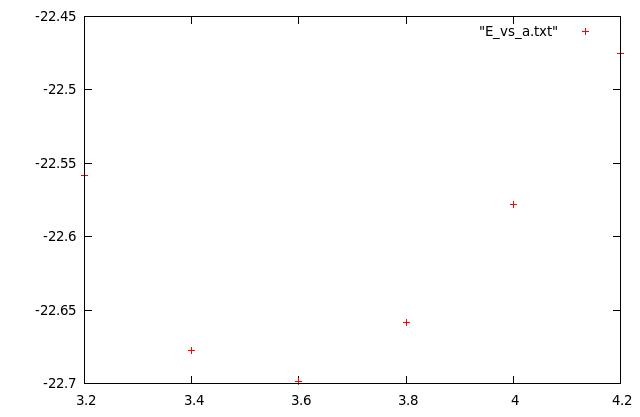Other than the ‘plot’, I’ve also written another one, fit for data sets just like these where you have a parabol-like data and you’d like to try fitting it and here is what it does (automatically):

```sururi@husniya:~/shared/qe/diamond_tutorial\$ plotfitparabol.sh E_vs_a.txt
func    f(x)=0.674197*x*x-4.881275*x-13.855234
min, f(min)    3.620066 -22.690502```Here are the source codes of the ‘plot’ and ‘plotfitparabol.sh’ scripts. Doei!

```sururi@mois:/vala/bin\$ cat plot
#!/bin/bash
gnuplot -persist <<END
set term postscript enhanced color
set output "\$1.ps"
plot "\$1" \$2 \$3

set term wxt
replot
END
sururi@mois:/vala/bin\$ cat plotfitparabol.sh
#!/bin/bash

# Usage : plotfitparabol.sh <datafile>

# Reads the data points from the given file
# then uses Octave's "polyfit" function to calculate the coefficients
# for the quadratic fit and also the minimum (maximum) value.
# Afterwards, prepares a GNUPlot script to display:
#    the data set (scatter)
#    fit function
#    the extremum point's value
#
# Written with Quantum Espresso optimizing procedures in mind.

if [ \$# -ne 1 ]
then
echo "Usage: plotfitparabol.sh <datafile>"
echo "Example: plotfitparabol.sh E_vs_A.txt"
echo -e "\nNeeds octave to be accessible via \"octave\" command to work"
echo -e "\nEmre S. Tasci <emre.tasci@ehu.es>, 03/2012"
exit
fi

oct=\$(echo \$octave_script|octave -q)
#echo \$oct

func=\${oct/|*/ }
minfmin=\${oct/*|/ }
echo -e "func \t" \$func
echo -e "min, f(min) \t" \$minfmin

echo '\$func
set term postscript enhanced color
set label "\$1" at graph 0.03, graph 0.94
set output "\$1.ps"
plot "\$1" title "data", f(x) title "\$func", "<echo '\$minfmin'" title "\$minfmin"

set term wxt
replot'

gnuplot -persist <<END
\$func
set term postscript enhanced color
set label "\$1" at graph 0.03, graph 0.94
set output "\$1.ps"
plot "\$1" title "data", f(x) title "\$func", "<echo '\$minfmin'" title "\$minfmin"

set term wxt
replot
END
```

Quantum Espresso meets bash : Primer and tidying up

Posted by Emre S. Tasci

In my previous postdoc position, DFT calculations were governing my research field but with my current position at the Bilbao Crystallographic Server, we are more on the theoretical side of things then calculations. Nevertheless, recently, my old ‘flame’ have been kindled and I decided to scrape the rust.

As a change, I selected and installed the Quantum Espresso (QE) package instead of VASP that I was using in the past, main reason QE being open source.

As a newbie to QE and long-gone returner to DFT, I started to practice by mostly ye olde trial/error approach.

The first thing that surprised me the lack of a commenting option in regard to QE input files! (Actually, I’m almost sure that it surely is supported but I have yet to find!). Here’s my sample input file:

```&control
title='Diamond Relaxing',

# Calculation Type ==========
calculation='scf'
#calculation='relax',
#calculation='vc-relax'
#calculation='cp'

# Restart ===================
restart_mode='from_scratch',
#restart_mode='restart'

#ndr = 51,
#ndw = 51,

#nstep = 100,
#nstep = 1000,
nstep = 2000,

# max_seconds = 600 # jobs stops after max_seconds CPU time.

iprint = 10, # band energies are written every iprint iterations
#tstress = .TRUE., # calculate stress. It is set to .TRUE. automatically if
# calculation='vc-md' or 'vc-relax'
#tprnfor = .TRUE., # print forces. Set to .TRUE. if calculation='relax','md','vc-md'
#dt = 5.0d0, # time step for molecular dynamics, in Rydberg atomic units
# (1 a.u.=4.8378 * 10^-17 s : beware, the CP code use
#  Hartree atomic units, half that much!!!)

prefix='diamond_rel', # prepended to input/output filenames
pseudo_dir = '/usr/share/espresso-4.3.2/pseudo/',
outdir='/home/sururi/shared/tmp/'
etot_conv_thr = 1.d-6 # convergence threshold on total energy (a.u) for ionic minimization
forc_conv_thr = 1.d-3 # convergence threshold on forces (a.u) for ionic minimization
/
&system
##  ibrav
##    0          "free", see above                 not used
##    1          cubic P (sc)                      not used
##    2          cubic F (fcc)                     not used
##    3          cubic I (bcc)                     not used
##    4          Hexagonal and Trigonal P        celldm(3)=c/a
##    5          Trigonal R, 3fold axis c        celldm(4)=cos(alpha)
##   -5          Trigonal R, 3fold axis &lt;111&gt;    celldm(4)=cos(alpha)
##    6          Tetragonal P (st)               celldm(3)=c/a
##    7          Tetragonal I (bct)              celldm(3)=c/a
##    8          Orthorhombic P                  celldm(2)=b/a,celldm(3)=c/a
##    9          Orthorhombic base-centered(bco) celldm(2)=b/a,celldm(3)=c/a
##   10          Orthorhombic face-centered      celldm(2)=b/a,celldm(3)=c/a
##   11          Orthorhombic body-centered      celldm(2)=b/a,celldm(3)=c/a
##   12          Monoclinic P, unique axis c     celldm(2)=b/a,celldm(3)=c/a,
##                                               celldm(4)=cos(ab)
##  -12          Monoclinic P, unique axis b     celldm(2)=b/a,celldm(3)=c/a,
##                                               celldm(5)=cos(ac)
##   13          Monoclinic base-centered        celldm(2)=b/a,celldm(3)=c/a,
##                                               celldm(4)=cos(ab)
##   14          Triclinic                       celldm(2)= b/a,
##                                               celldm(3)= c/a,
##                                               celldm(4)= cos(bc),
##                                               celldm(5)= cos(ac),
##                                               celldm(6)= cos(ab
ibrav= 2,

# Cell dimensions ========================
# a,b,c in Angstrom =======
A = 3.56712
B = 2.49
C = 2.49
cosAB=0.5
cosAC=0.5
cosBC=0.5

## OR ##
## celldm(1:6) in Bohr =====
## 1 Bohr =  0.529177249 A
## 1 A = 1.889725989 Bohr
# celldm(1) = 6.7409 # alat
# celldm(2) = 4.7054 # b/a
# celldm(3) = 4.7054 # c/a
# celldm(4) = 0.5 # cos(ab)
# celldm(5) = 0.5 # cos(ac)
# celldm(6) = 0.5 # cos(ab)

nat=2,
ntyp= 1,

ecutwfc = 40, # kinetic energy cutoff (Ry) for wavefunctions
# ecutrho = 160 # kinetic energy cutoff (Ry) for charge density and potential.
# For norm-conserving pseudopotential you should stick to the
# default value
# nbnd = 6 # number of electronic states (bands) to be calculated.
# Default:
# for an insulator, nbnd = number of valence bands (nbnd = # of electrons /2);
# for a metal, 20% more (minimum 4 more)
/
&electrons
emass = 400.d0
emass_cutoff = 2.5d0

#electron_dynamics = 'sd' # CP specific
#electron_dynamics = 'bfgs' # CP specific

conv_thr = 1D-6 # Convergence threshold for selfconsistency

/
&ions
#ion_dynamics = 'none'
#ion_dynamics = 'bfgs'
#ion_dynamics = 'sd'

# === damping === 0 ==
# ion_dynamics = 'damp'
# ion_damping = 0.2
# ion_velocities = 'zero' # CP specific -- initial ionic velocities
# === damping === 1 ==

#ion_nstepe = 10 # CP specific -- number of electronic steps per ionic step. (Def: 1)
/
&cell
cell_dynamics = 'none',
#cell_dynamics = 'bfgs', # ion_dynamics must be 'bfgs' too
#cell_dofree = 'xyz'
#cell_factor = 3
press = 0.0d,
# press_conv_thr = 0.05 # Convergence threshold on the pressure for variable cell relaxation
/
ATOMIC_SPECIES
C  12.0107 C.pz-vbc.UPF
ATOMIC_POSITIONS
C 0.0 0.0 0.0
C 0.25 0.25 0.25
K_POINTS automatic
4 4 4 1 1 1```

so, it’s not the tidiest parameter file around but nevertheless, I’ve included some explanations and it’s easily customizable by simple commenting out, right?…

And then the problem of (yet undiscovered) comments in the QE input files hits us. But with bash, it’s pretty easy. So, if you save the above contents to a file called, say, “diamond.scf.in” and filter it via grep and sed, you’re done:

```sururi@husniya:~/shared/qe/diamond_tutorial\$ cat diamond.scf.in | grep -v -E "^[\t ]*#" | sed "s:\([^#]*\)#.*:\1:"
&control
title='Diamond Relaxing',

calculation='scf'

restart_mode='from_scratch',

nstep = 2000,

iprint = 10,

prefix='diamond_rel',
pseudo_dir = '/usr/share/espresso-4.3.2/pseudo/',
outdir='/home/sururi/shared/tmp/'
etot_conv_thr = 1.d-6
forc_conv_thr = 1.d-3
/
&system
ibrav= 2,

A = 3.56712
B = 2.49
C = 2.49
cosAB=0.5
cosAC=0.5
cosBC=0.5

nat=2,
ntyp= 1,

ecutwfc = 40,
/
&electrons
emass = 400.d0
emass_cutoff = 2.5d0

conv_thr = 1D-6

/
&ions

/
&cell
cell_dynamics = 'none',
press = 0.0d,
/
ATOMIC_SPECIES
C  12.0107 C.pz-vbc.UPF
ATOMIC_POSITIONS
C 0.0 0.0 0.0
C 0.25 0.25 0.25
K_POINTS automatic
4 4 4 1 1 1```

I’ve installed the QE to take advantage of the cluster nodes via MPI, so I submit my jobs via:
`mpirun -n #_of_processes /usr/share/espresso-4.3.2/bin/pw.x < input_file > output_file`

which I guess is more or less similar to what you are doing to run your jobs.

So, combining this “comment filtering” and job submission, I’ve written the following bash script (“mpi-pw.x“) to automatize (and keep log of) the job:

``````sururi@cowboy:~\$ cat /home/sururi/bin/mpi-pw.x
#!/bin/bash

# Script to submit Quantum Espresso jobs via mpi
# EST, Fri Mar  9 17:02:10 CET 2012

function timer()
{
if [[ \$# -eq 0 ]]; then
echo \$(date '+%s')
else
local  stime=\$1
etime=\$(date '+%s')

if [[ -z "\$stime" ]]; then stime=\$etime; fi

dt=\$((etime - stime))
ds=\$((dt % 60))
dm=\$(((dt / 60) % 60))
dh=\$((dt / 3600))
printf '%d:%02d:%02d' \$dh \$dm \$ds
fi
}

if [ \$# -ne 2 ]
then
echo "Usage: mpi-pw.x num_of_processes infile.in"
exit
fi

job=\$2
job_wo_ext=\${job%.*}

outext=".out"
logext=".log"
echo -e "Running: mpirun -n \$1 /usr/share/espresso-4.3.2/bin/pw.x < \$2 > \$job_wo_ext\$outext\n"
echo -e "Running: mpirun -n \$1 /usr/share/espresso-4.3.2/bin/pw.x < \$2 > \$job_wo_ext\$outext\n">\$job_wo_ext\$logext
echo "Start : "\$(date)
echo "Start : "\$(date)>>\$job_wo_ext\$logext

# Take out the comments from the input file
#grep -v -E "^[\t ]*#" \$2 > in_wo_comments.in.tmp
grep -v -E "^[\t ]*#" \$2 | sed "s:\([^#]*\)#.*:\1:" > in_wo_comments.in.tmp

t=\$(timer)
mpirun -n \$1 /usr/share/espresso-4.3.2/bin/pw.x < in_wo_comments.in.tmp > \$job_wo_ext\$outext
echo "Finish: "\$(date)
echo "Finish: "\$(date)>>\$job_wo_ext\$logext
printf 'Elapsed time:%s\n\n' \$(timer \$t)
printf 'Elapsed time:%s\n\n' \$(timer \$t) >>\$job_wo_ext\$logext

(I’ve picked the “timer” function from Mitch Frazier’s entry in LinuxJournal)

What it does is, really simple: it strips out the extension of the input file (\$2 – the second argument in calling); filters out the comments, constructing the temporary input file “in_wo_comments.in.tmp”; starts the timer; submits the job using the number of processes passed in the calling (\$1 – first argument) and directs the output to a file whose name is the extension stripped input filename + “.out”; when the job is (this way or that) done, stops the timer and calculates the elapsed time via the ‘timer’ function. During all this time, it prints these information both on screen and to a file called extension stripped input filename + “.log”.

I have a very similar another script called “mpi-cp.x” that I’ve forked for Carr-Parinelli jobs (just search & replace all the occurrences of “mpi-pw.x” with “mpi-cp.x” and you’re done 8).

And here is the script in action:

```sururi@cowboy:~/shared/qe/diamond_tutorial\$ ll
total 16
drwxr-xr-x 2 sururi sururi 4096 2012-03-13 15:59 ./
drwxr-xr-x 7 sururi sururi 4096 2012-03-13 15:10 ../
-rw-r--r-- 1 sururi sururi 4954 2012-03-13 15:59 diamond.scf.in

sururi@cowboy:~/shared/qe/diamond_tutorial\$ mpi-pw.x 4 diamond.scf.in
Running: mpirun -n 4 /usr/share/espresso-4.3.2/bin/pw.x < diamond.scf.in > diamond.scf.out

Start : Tue Mar 13 16:18:20 CET 2012
Finish: Tue Mar 13 16:18:21 CET 2012
Elapsed time:0:00:01

sururi@cowboy:~/shared/qe/diamond_tutorial\$ grep -e ! diamond.scf.out
!    total energy              =     -22.70063050 Ry

sururi@cowboy:~/shared/qe/diamond_tutorial\$ cat diamond.scf.log
Running: mpirun -n 4 /usr/share/espresso-4.3.2/bin/pw.x < diamond.scf.in > diamond.scf.out

Start : Tue Mar 13 16:18:20 CET 2012
Finish: Tue Mar 13 16:18:21 CET 2012
Elapsed time:0:00:01```

Now that we have the mpi-pw.x and mpi-cp.x, our next entry in the series will be the automated search for optimized values.